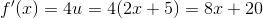# High School Math : Using the Chain Rule

## Example Questions

### Example Question #1 : Using The Chain Rule

If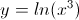what is the slope of the line at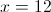.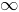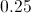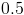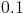Explanation:

The slope at any point on a line is also equal to the derivative. So first we want to find the derivative function of this function and then evaluate it at. So, to find the derivative we will need to use the chain rule. The chain rule says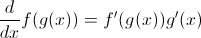so if we let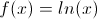and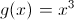then

since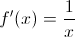and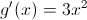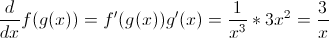Therefore we evaluate atand we get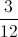or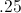.

### Example Question #2 : Using The Chain Rule

What is the first derivative of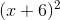?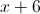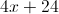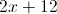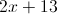Explanation:

To solve for the first derivative, we're going to use the chain rule. The chain rule says that when taking the derivative of a nested function, your answer is the derivative of the outside times the derivative of the inside.

Mathematically, it would look like this: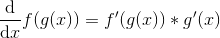Plug in our equations.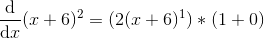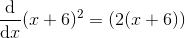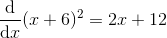### Example Question #1 : Using The Chain Rule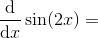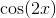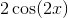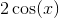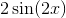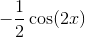Explanation:

For this problem we need to use the chain rule: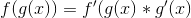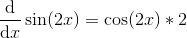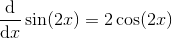### Example Question #71 : Calculus I — Derivatives

Find the derivative of the following function: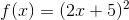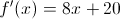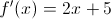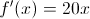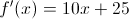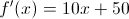Explanation:

Use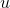-substitution so that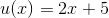.

Then the functionbecomes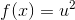.

By the chain rule,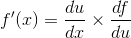.

We calculate each term using the power rule: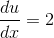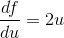Plug in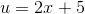: